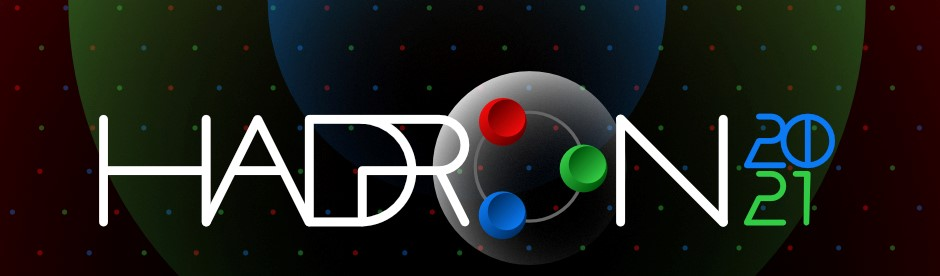19th International Conference on Hadron Spectroscopy and Structure in memoriam Simon Eidelman

26-31 July 2021
Mexico City
Mexico/General timezone
HADRON 2021 is over. Thanks for making it a success!
Home > Timetable > Session details > Contribution details

Mexico City -

Are the $Z_{cs}(3985)$ and $Z_{cs}(4000)$ the same state?

• Dr. Lu MENG

Abstract

In this talk, I will provide distinguishable consequences of the $Z_{cs}(3985)$ and $Z_{cs}(4000)$ as two or one state. In the one-state scheme, $Z_{cs}(3985/4000)^−$ state is the U-spin partner of $Z_c(3900)^−$. Otherwise, the SU(3) flavor partner of $Z_{c}(3900)$ is $Z_{cs}(4000)$ rather than the $Z_{cs}(3985)$. Another two important predictions in the two-state scheme are the existence of a tensor $barD_s^∗D^∗$ resonance and suppression of decay mode $Z_{cs}(3985) \to J/\psi K$.